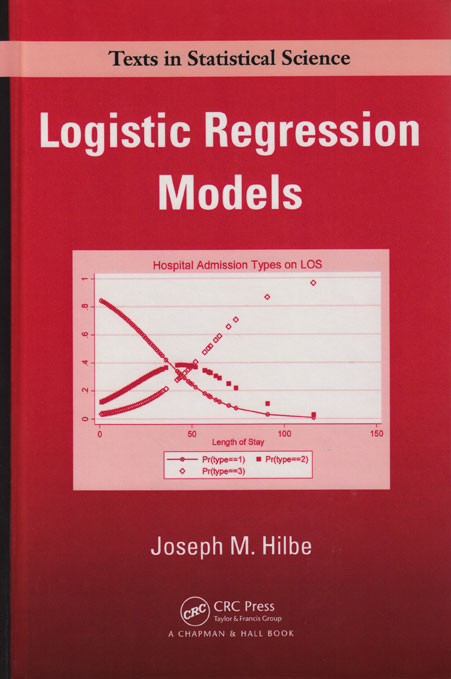# Logistic Regression Models

Logistic Regression Models, by Joseph Hilbe, arose from Hilbe’s course in logistic regression at statistics.com. The book includes many Stata examples using both official and user-written commands and includes Stata output and graphs.

Hilbe begins with simple contingency tables and covers fitting algorithms, parameter interpretation, and diagnostics. The later chapters include models for overdispersion, complex response variables, longitudinal data, and survey data. The final chapter describes exact logistic regression, available in Stata 10 with the new exlogistic command. Hilbe does not oversimplify controversial issues, like interactions and standardized coefficients.

The prerequisite for most of the book is a working knowledge of multiple regression, but some sections use multivariate calculus and matrix algebra.

Hilbe is coauthor (with James Hardin) of the popular Stata Press book Generalized Linear Models and Extensions. He also wrote the first versions of Stata’s logistic and glm commands.

The fourth printing has been revised: examples in the book now use Stata version 11 code in place of earlier version code, where applicable.

Preface

CHAPTER 1 INTRODUCTION

The Normal Model
Foundation of the Binomial Model
Historical and Software Considerations
Chapter Profiles

CHAPTER 2 CONCEPTS RELATED TO THE LOGISTIC MODEL

2 × 2 Table Logistic Model
2 × k Table Logistic Model
Modeling a Quantitative Predictor
Logistic Modeling Designs

Experimental Studies
Observational Studies

Prospective or Cohort Studies
Retrospective or Case–Control Studies
Comparisons

Exercises
R Code

CHAPTER 3 ESTIMATION METHODS

Derivation of the IRLS Algorithm
IRLS Estimation
Maximum Likelihood Estimation

Exercises
R Code

CHAPTER 4 DERIVATION OF THE BINARY LOGISTIC ALGORITHM

Terms of the Algorithm
Logistic GLM and ML Algorithms
Other Bernoulli Models
Exercises
R Code

CHAPTER 5 MODEL DEVELOPMENT

Building a Logistic Model

Interpretations
Full Model
Reduced Model

Box–Tidwell Test
Test by Partial Residuals
Linearity of Slopes Test
Fractional Polynomials

Standardized Coefficients
Standard Errors

Calculating Standard Errors
The z-Statistic
p-Values
Confidence Intervals
Confidence Intervals of Odds Ratios

Odds Ratios as Approximations of Risk Ratios

Epidemiological Terms and Studies
Odds Ratios, Risk Ratios, and Risk Models
Calculating Standard Errors and Confidence Intervals
Risk Difference and Attributable Risk
Other Resources on Odds Ratios and Risk Ratios

Scaling of Standard Errors
Robust Variance Estimators
Bootstrapped and Jackknifed Standard Errors
Stepwise Methods
Handling Missing Values
Modeling an Uncertain Response
Constraining Coefficients
Exercises
R Code

CHAPTER 6 INTERACTIONS

Introduction
Binary × Binary Interactions

Interpretation—as Odds Ratio
Standard Errors and Confidence Intervals
Graphical Analysis

Binary × Categorical Interactions
Binary × Continuous Interactions

Notes on Centering
Constructing and Interpreting the Interaction
Interpretation
Standard Errors and Confidence Intervals
Significance of Interaction
Graphical Analysis

Categorical × Continuous Interactions

Interpretation
Standard Errors and Confidence Intervals
Graphical Representation

Binary × Binary
Continuous × Binary
Continuous × Continuous

Exercises
R Code

CHAPTER 7 ANALYSIS OF MODEL FIT

Traditional Fit Tests for Logistic Regression

R2 and Pseudo-R2 Statistics
Deviance Statistic
Likelihood Ratio Test

Hosmer–Lemeshow GOF Test

Hosmer–Lemeshow GOF Test
Classification Matrix
ROC Analysis

Information Criteria Tests

Akaike Information Criterion—AIC
Finite Sample AIC Statistic
LIMDEP AIC
SWARTZ AIC
Bayesian Information Criterion (BIC)
HQIC Goodness-of-Fit Statistic
A Unified AIC Fit Statistic

Residual Analysis

GLM-Based Residuals

Raw Residual
Pearson Residual
Deviance Residual
Standardized Pearson Residual
Standardized Deviance Residual
Likelihood Residuals
Anscombe Residuals

m-Asymptotic Residuals

Hat Matrix Diagonal Revisited
Other Influence Residuals

Conditional Effects Plot

Validation Models
Exercises
R Code

CHAPTER 8 BINOMIAL LOGISTIC REGRESSION

Exercises
R Code

CHAPTER 9 OVERDISPERSION

Introduction
The Nature and Scope of Overdispersion
Binomial Overdispersion

Apparent Overdispersion

Simulated Model Setup
Missing Predictor
Needed Interaction
Predictor Transformation
Existing Outlier(s)

Relationship: Binomial and Poisson

Binary Overdispersion

The Meaning of Binary Model Overdispersion
Implicit Overdispersion

Real Overdispersion

Methods of Handling Real Overdispersion
Williams’ Procedure
Generalized Binomial Regression

Concluding Remarks

Exercises
R Code

CHAPTER 10 ORDERED LOGISTIC REGRESSION

Introduction
The Proportional Odds Model
Generalized Ordinal Logistic Regression
Partial Proportional Odds

Exercises
R Code

CHAPTER 11 MULTINOMIAL LOGISTIC REGRESSION

Unordered Logistic Regression

The Multinomial Distribution
Interpretation of the Multinomial Model

Independence of Irrelevant Alternatives

Comparison to Multinomial Probit
Exercises
R Code

CHAPTER 12 ALTERNATIVE CATEGORICAL RESPONSE MODELS

Introduction
Continuation Ratio Models
Stereotype Logistic Model
Heterogeneous Choice Logistic Model
Proportional Slopes Models

Proportional Slopes Comparative Algorithms
Modeling Synthetic Data
Tests of Proportionality

Exercises

CHAPTER 13 PANEL MODELS

Introduction
Generalized Estimating Equations

GEE: Overview of GEE Theory
GEE Correlation Structures

Independence Correlation Structure Schematic
Exchangeable Correlation Structure Schematic
Autoregressive Correlation Structure Schematic
Unstructured Correlation Structure Schematic
Stationary or m-Dependent Correlation Structure Schematic
Nonstationary Correlation Structure Schematic

GEE Binomial Logistic Models
GEE Fit Analysis—QIC

QIC/QICu Summary–Binary Logistic Regression

Alternating Logistic Regression
Quasi-Least Squares Regression
Feasibility

Unconditional Fixed Effects Logistic Model
Conditional Logistic Models

Conditional Fixed Effects Logistic Models
Matched Case–Control Logistic Model
Rank-Ordered Logistic Regression

Random Effects and Mixed Models Logistic Regression

Random Effects and Mixed Models: Binary Response
Alternative AIC-Type Statistics for Panel Data
Random-Intercept Proportional Odds

Exercises
R Code

CHAPTER 14 OTHER TYPES OF LOGISTIC-BASED MODELS

Survey Logistic Models

Interpretation

Scobit-Skewed Logistic Regression
Discriminant Analysis

Dichotomous Discriminant Analysis
Canonical Linear Discriminant Analysis
Linear Logistic Discriminant Analysis

Exercises

CHAPTER 15 EXACT LOGISTIC REGRESSION

Exact Methods
Alternative Modeling Methods

Monte Carlo Sampling Methods
Median Unbiased Estimation
Penalized Logistic Regression

Exercises
Conclusion

APPENDIX A: Brief Guide to Using Stata Commands

APPENDIX B: Stata and R Logistic Models

APPENDIX C: Greek Letters and Major Functions

APPENDIX D: Stata Binary Logistic Command

APPENDIX E: Derivation of the Beta Binomial

APPENDIX F: Likelihood Function of the Adaptive Gauss–Hermite Quadrature Method of Estimation

APPENDIX G: Data Sets

APPENDIX H: Marginal Effects and Discrete ChangeAuthor: Joseph M. Hilbe
ISBN-13: 978-1-4200-7575-5